1
120
views
6
Problem

Problem 6

Chapter 1: Functions and ModelsTextbook ExpertVerified Tutor
18 Oct 2021

Given information

The given is:

The linear function is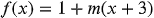.

Step-by-step explanation

Step 1.

Explanation:

Recall the fact that the slope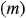of the function is the coefficient of the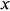term and the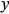intercept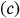is the constant term of the function.